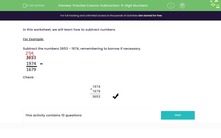# Practise Column Subtraction of Four-Digit Numbers

In this worksheet, students will subtract four-digit numbers using columns with borrowing if necessary.Key stage:  KS 2

Curriculum topic:   Number: Addition and Subtraction

Curriculum subtopic:   Add/Subtract up to Four-Digit Numbers

Popular topics:   Subtraction worksheets

Difficulty level:#### Worksheet Overview

In this activity, we will practise how to subtract numbers using borrowing.

Example:

Subtract the numbers 3,653 - 1,974, remembering to borrow if necessary.The important thing to remember about using column method for subtraction is that we must be careful to line up the numbers with the digits in their correct place. Then, we always start at the right, with the ones.

Let's have a go at some questions now, but remember that you can check back to this example at any point by clicking on the red help button that appears on the screen when you start the questions.